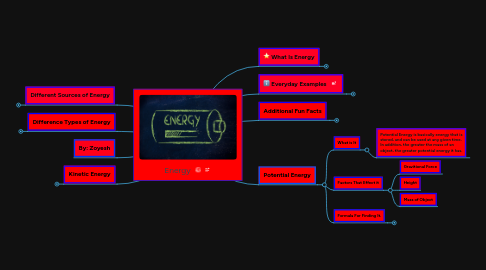# EnergyZoyesh IRFAN

Get Started. It's FreeEnergy## 2. Everyday Examples

### 2.1. Motion Energy

2.1.1. Car in motion

2.1.2. Flying Plane

2.1.3. Bullet

### 2.2. Thermal Energy

2.2.1. Boiling water

2.2.2. Coffee/Tea

### 2.3. Sound Energy

2.3.1. Dog Barking

2.3.2. Shouting

2.3.3. Car Engine

## 3. Difference Types of Energy

### 3.1. Potential Energy

3.1.1. Nuclear Energy

3.1.2. Chemical Energy

3.1.3. Gravitational Energy

### 3.2. Kinetic Energy

3.2.1. Thermal Energy

3.2.3. Electric Energy

## 7. Kinetic Energy

### 7.1. What is it?

7.1.1. Kinetic Energy is the energy an object possess in order to have motion

### 7.2. Variables/Factors it Depends on

7.2.1. There are two main variables involved in the translation of kinetic energy: speed and mass. If more kinetic energy is applied, then the object will go at a faster rate,

### 7.4. Formula For finding kinetic energy

7.4.1. KE= 0.5 * m * v

## 8. Potential Energy

### 8.1. What is It

8.1.1. Potential Energy is basically energy that is stored, and can be used at any given time. In addition, the greater the mass of an object, the greater potential energy it has.

### 8.2. Factors That Effect it

8.2.1. Gravitional Force

8.2.2. Height

8.2.3. Mass of Object

### 8.3. Formula For Finding It.

8.3.1. PEgrv = m * g * h